# Flowerbeds

What is the smallest length of flowerbeds we have to prepare to plant seedlings at grid 20,30,25,40 cm?

x =  600 cm

### Step-by-step explanation: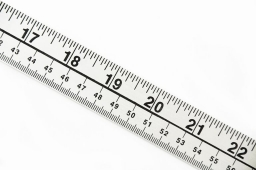Did you find an error or inaccuracy? Feel free to write us. Thank you!Tips to related online calculators
Do you want to calculate least common multiple two or more numbers?
Do you want to convert length units?

## Related math problems and questions:

• Gcd and lcm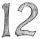Calculate the greatest common divisor and the least common multiple of numbers. a) 16 and 18 b) 24 and 22 c) 45 and 60 d) 36 and 30
• LCMWhat is the least common multiple of 5, 50, 14?
• Lcm of three numbersWhat is the Lcm of 120 15 and 5
• TilesHow many tiles of 20 cm and 30 cm can build a square if we have a maximum of 100 tiles?
• A ropeA rope can be cut into equal length with no rope left over. The lengths can be 15cm,18cm or 25cm. What is the shortest possible length of the rope?
• Lcm simpleFind least common multiple of this two numbers: 140 175.
• CubesCarol with cut bar 12 cm x 12 cm x 135 cm to the cubes. Find the sum of all the surfaces of the resulting cubes.
• LCM of two numberFind the smallest multiple of 63 and 147
• Square roomWhat is the size of the smallest square room, which can pave with tiles with dimensions 55 cm and 45 cm? How many such tiles is needed?
• Two rulers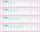We have two rulers. Scale interval on first rulers are a spaced 1 cm and on second spaced 15 mm. Rulers are attached to each other so that they match initial divider commas. What next dividers commas coincide? Find at least three cases.
• Steps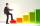Peter makes steps long 70 cm, John 45 cm long. After how many meters their footsteps meet?
• Bus linesAt 5.00 in the morning, four buses left the terminal at the same time. The first bus has an interval of 15 minutes, the second 20 minutes, the third 25 minutes, and the fourth 45 minutes. How many minutes will all four buses leave the terminal at the same
• Counting numberWhat is the smallest counting number divisible by 2,5,7,8, and 15?
• PexesoDrawing paper has dimensions of 60cm and 840mm. Pupils have to divide it into squares so that they can make a pexeso. What dimension must squares have if their side is to be larger than 3cm and less than 10cm?
• DecomposeDecompose into primes and find the smallest common multiple n of (16,20) and the largest common divisor D of the pair of numbers (140,100)
• The smallest numberWhat is the smallest number that can be divided by both 5 and 7
• 3 buses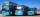At morning 5:00 am three buses started from one place. The first travel in five-minute intervals, the second at 10-minute intervals and the third at 25-minute intervals. At what hour will again be the three buses coming from the same place?